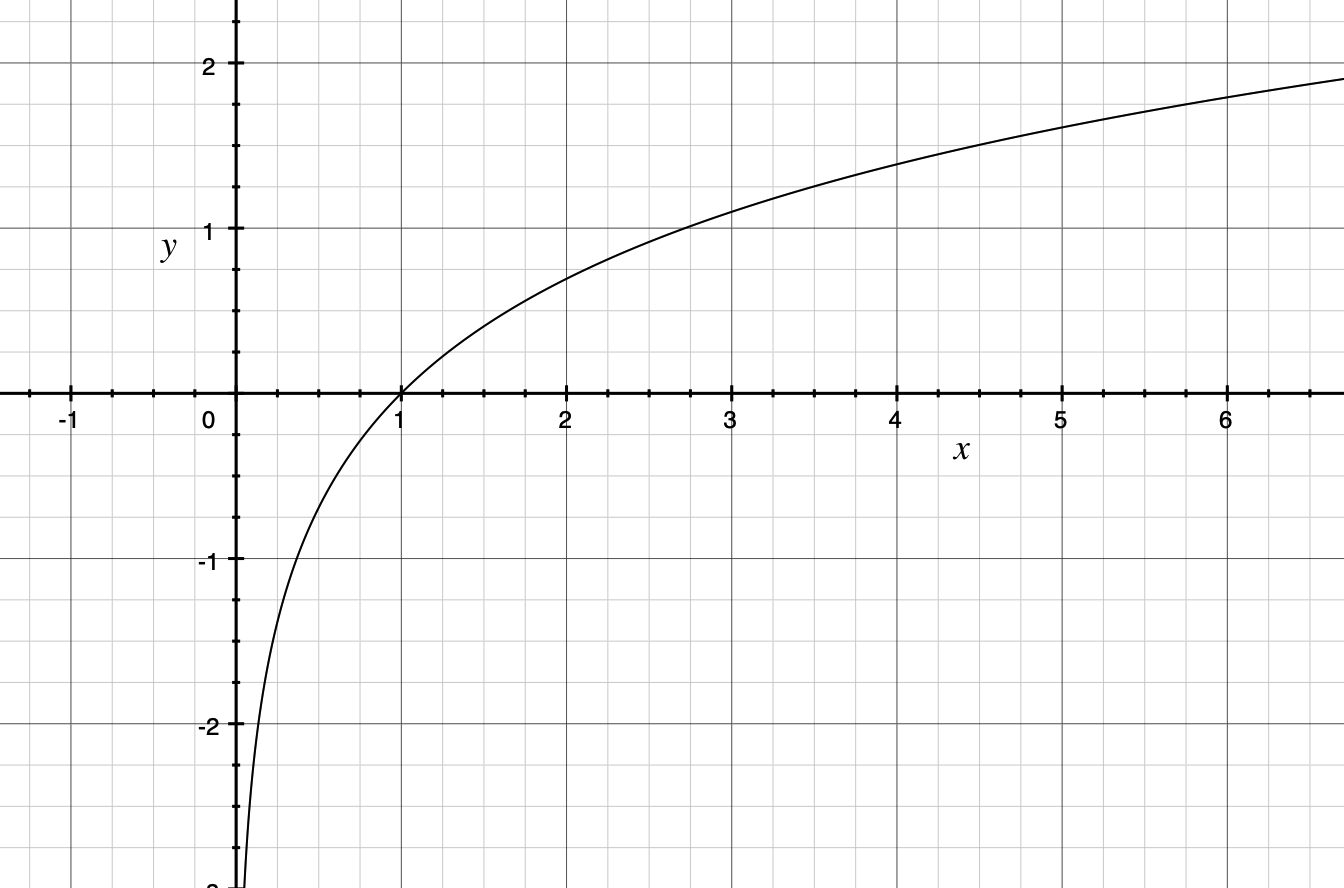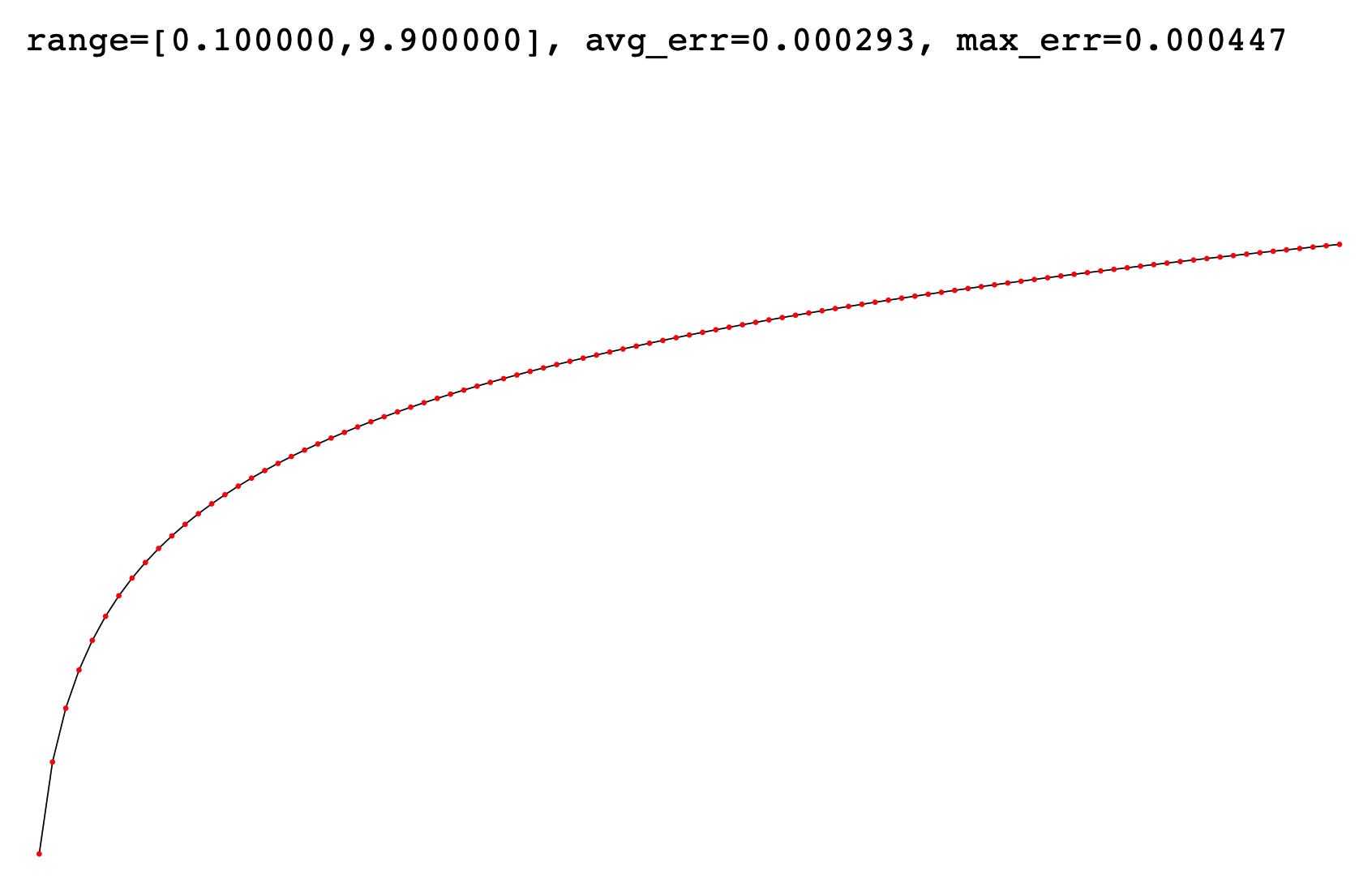# Fast Natural Log Approximation

— with Evil Bit Hacking and Remez Polynomials

2021/03/02

In one of my projects, where the math standard library is not available, I needed really simple, but fast approximations for cos, exp and log functions. I found great snippets on the Internet for the first two, but had to combine several inspirations and come up with my own solution for the natural log.

First, the final code:

float ln(float x) {
unsigned int bx = * (unsigned int *) (&x);
unsigned int ex = bx >> 23;
signed int t = (signed int)ex-(signed int)127;
unsigned int s = (t < 0) ? (-t) : t;
bx = 1065353216 | (bx & 8388607);
x = * (float *) (&bx);
return -1.49278+(2.11263+(-0.729104+0.10969*x)*x)*x+0.6931471806*t;
}


I tested it to be over thrice as fast as <math.h>'s log() under gcc -O3 on my mac laptop.

Here's a graph of $\ln{x}$, which I found useful to stare at while solving the problem:Here's how I came up with the algorithm:

First recall that high school taught us the Taylor Series for natural logarithm is

$\ln(1+x)=x-\frac{x^2}{2}+\frac{x^3}{3}-...$

But it turns out nobody is seriously using Taylor Series for coding math functions because calculating exponents are slow and it takes many terms to converge.

This SO answer pointed me to the Remez algorithm, which I didn't fully understand, but the simplicity and accuracy of the approximation do seem very nice.

They only gave the 4th order approximation for the range [1,2] in the answer:

$-1.7417939 + (2.8212026 + (-1.4699568 + (0.44717955 - 0.056570851 x) x) x) x$

However I'd like to try figure out the 3rd order too, since in my application I can do with a little bit less accuracy (and more speed). I don't have Maple software mentioned by the answer, but I found this blog post where the author computed approximation for atan2 with Boost, the C++ library's built-in function.

It turns out Boost removed the function (along with other “useless” math tools) in their latest versions, and I had to install an older version. Then simply invoke the function like so:

boost::math::tools::remez_minimax<double> approx(
[](const double& x) { return log(x); },
4, 0, 1, 2, false, 0, 0, 64);


And find the constants for the 3rd order approximation to be:

{ -1.49278, 2.11263, -0.729104, 0.10969 }


And therefore

$-1.49278+(2.11263+(-0.729104+0.10969x)x)x$

However this is only solving half of the problem, because the approximation is only good for the range [1,2].

Luckily, this helpful site points out the formula

If

$x = m \cdot 10^p$

then

$\ln{x} = \ln{m} + 2.3025851 p$

which basically says that if you keep dividing the number in question by 10, until it's between 1 and 10, and calculate the natural log for that number, you can infer the natural log for the original number by a simple multiplication and addition.

Now one way is to compute the Remez approximation for the range [1,10] (which is intuitively less accurate given the same number of terms), but it's easy to realize that the formula above works for base 2 too. And in fact, using base 2 gives us a huge advantage due to representation of floating points on computer systems, which we'll see very soon.

So we have this modified formula which works for range [1,2]:

If

$x = m \cdot 2^p$

then

$\ln{x} = \ln{m} + 0.6931471806 p$

The final question is how do we get the original number into the range [1,2] (while remembering the number of 2s we took from it). The naive solution is to use while loops with a counter, like so:

int p = 0;
while (x > 2){
x /= 2;
++p;
}
while (x < 1){
x *= 2;
--p;
}


But that sure doesn't look too efficient!

And this is one of those moments when my CS education unexpectedly pay off. Recall that an IEEE 32-bit float is stored in memory like so:

   sign  exp       frac
0b 0     00000000  00000000000000000000000


The idea behind this representation is quite like that of the scientific notation. We keep track of the first couple significant figures, and also the exponent we need to raise them to.

Here we assume that the number is the non-denormalized form, i.e. $x > 2^{-126}$. Which is a valid assumption, because otherwise $\ln{x}$ approaches negative infinity anyways.

So to get the value of the float (for humans), we have the formula:

$x = (-1)^s * M * 2 ^{exp-127}$

By comparing the formulae, it is easy to spot that exp-127 is exactly the $p$ we needed for $x = m \cdot 2^p$.

To extract the exp bits, we can simply right shift by 23. Since logarithm is only defined for positive numbers, we don't need to worry about masking out the sign bit.

Therefore, in the spirit of “evil floating point bit level hacking” from Q_rsqrt, we have:

unsigned int bx = * (unsigned int *) (&x);
unsigned int ex = bx >> 23;
signed int p = (signed int)ex-(signed int)127;


float natural_log(float x) {

// ASSUMING:
// - non-denormalized numbers i.e. x > 2^-126
// - integer is 32 bit. float is IEEE 32 bit.

// INSPIRED BY:
// - https://stackoverflow.com/a/44232045
// - http://mathonweb.com/help_ebook/html/algorithms.htm#ln
// - https://en.wikipedia.org/wiki/Fast_inverse_square_root

// FORMULA:
// x = m * 2^p =>
//   ln(x) = ln(m) + ln(2)p,

// first normalize the value to between 1.0 and 2.0
// assuming normalized IEEE float
//    sign  exp       frac
// 0b 0     00000000000000000000000
// value = (-1)^s * M * 2 ^ (exp-127)
//
// exp = 127 for x = 1,
// so 2^(exp-127) is the multiplier

// evil floating point bit level hacking
unsigned int bx = * (unsigned int *) (&x);

// extract exp, since x>0, sign bit must be 0
unsigned int ex = bx >> 23;
signed int t = (signed int)ex-(signed int)127;
unsigned int s = (t < 0) ? (-t) : t;

// reinterpret back to float
//   127 << 23 = 1065353216
//   0b11111111111111111111111 = 8388607
bx = 1065353216 | (bx & 8388607);
x = * (float *) (&bx);

// use remez algorithm to find approximation between [1,2]
// - see this answer https://stackoverflow.com/a/44232045
// - or this usage of C++/boost's remez implementation
//   https://computingandrecording.wordpress.com/2017/04/24/
// e.g.
// boost::math::tools::remez_minimax<double> approx(
//    [](const double& x) { return log(x); },
// 4, 0, 1, 2, false, 0, 0, 64);
//
// 4th order is:
// { -1.74178, 2.82117, -1.46994, 0.447178, -0.0565717 }
//
// 3rd order is:
// { -1.49278, 2.11263, -0.729104, 0.10969 }

return

/* less accurate */
-1.49278+(2.11263+(-0.729104+0.10969*x)*x)*x

/* OR more accurate */
// -1.7417939+(2.8212026+(-1.4699568+(0.44717955-0.056570851*x)*x)*x)*x

/* compensate for the ln(2)s. ln(2)=0.6931471806 */
+ 0.6931471806*t;
}


You can find the full source code on Github Gist here.

Because in fact I need the algorithm in WebAssembly and not in C for my use case, here's the handwritten WAT (WebAssembly Text format) port for anyone interested:

;; natural log ln(x) approximation
(func $log (param$x f32) (result f32)

(local $bx i32) (local$ex i32)
(local $t i32) (local$s  i32)
(local.set $bx (i32.reinterpret_f32 (local.get$x)))
(local.set $ex (i32.shr_u (local.get$bx) (i32.const 23)))
(local.set $t (i32.sub (local.get$ex) (i32.const 127)))
(local.set $s (local.get$t))
(if (i32.lt_s (local.get $t) (i32.const 0)) (then (local.set$s (i32.sub (i32.const 0) (local.get $t) )) )) (local.set$bx (i32.or
(i32.const 1065353216)
(i32.and (local.get $bx) (i32.const 8388607) ) )) (local.set$x (f32.reinterpret_i32 (local.get $bx) )) (f32.add (f32.add (f32.const -1.49278) (f32.mul (f32.add (f32.const 2.11263) (f32.mul (f32.add (f32.const -0.729104) (f32.mul (f32.const 0.10969) (local.get$x)))
(local.get $x))) (local.get$x)))

(f32.mul
(f32.convert_i32_s (local.get \$t))
(f32.const 0.6931471806)
)
)
)


## Testing Accuracy and Speed

To evaluate the accuracy of the algorithm, we can use the following code, which prints out a SVG plotting graph of our ln() alongside <math.h>'s log():

float X = 10;
float Y = 4;
float step = 0.1;
float scl = 5;
int main() {
printf("<svg version=\"1.1\" xmlns=\"http://www.w3.org/2000/svg\" width=\"%f\" height=\"%f\">\n",X/step*scl,Y/step*2*scl);
printf("<path fill=\"none\" stroke=\"black\" stroke-width=\"0.5\" d=\"M ");
for (float x = step; x < X; x+=step){
float y = log(x);
y = (Y - y ) /step;
printf("%f %f ",x/step*scl,y*scl);
}
printf("\" />\n");
float dy = 0;
float max_dy = 0;
int cnt = 0;
for (float x = step; x < X; x+=step){
float y0 = log(x);
float y = ln(x);
max_dy = fmax(fabs(y0-y),max_dy);
dy += fabs(y0-y); cnt ++;
y = (Y - y ) /step;
printf("<circle cx=\"%f\" cy=\"%f\" r=\"1\" fill=\"red\" />\n",x/step*scl,y*scl);
}
float avg_dy = dy/(float)cnt;
printf("<text font-family=\"monospace\" x=\"0\" y=\"12\">range=[%f,%f], avg_err=%f, max_err=%f</text>\n",step,X-step,avg_dy,max_dy);
printf("</svg>\n");
return 0;
}


Compile, and produce the SVG file by redirecting stdout to file:

gcc -O3 ln.c ; ./a.out > ./a.svg


You'll be able to see the <math.h>'s log() plotted as a black line, and our ln() as a series of red dots; the (absolute) average error and maximum error are also displayed.▲ The error is too tiny for human eyes to observe from a plot

For the small image above, we used a largish step and a smallish range, but you can try out the testing code with different parameters to see pretty much identical reports on accuracy.

If we use the 4th order polynomial in place of the 3rd, i.e.

return -1.7417939+(2.8212026+(-1.4699568+(0.44717955-0.056570851*x)*x)*x)*x+0.6931471806*t;


We'll be able to even trim down the (absolute) error to:

avg_err=0.000039, max_err=0.000061


Yup! That's four zeros after the decimal point.

Now to test the speed of the algorithm, we can use this code below:

#include <sys/time.h>
#include <stdlib.h>

int main(){
srand(time(NULL));
float* ans = malloc(sizeof(float)*100);
unsigned int N = 1000000000;

{
struct timeval t0, t1;
unsigned int i;
gettimeofday(&t0, NULL);
for(i = 0; i < N; i++){
float y = log((float)i/1000.0);
ans[i%100]=y;
}
gettimeofday(&t1, NULL);
printf("%u <math.h> log() calls in %.10f seconds\n", i, t1.tv_sec - t0.tv_sec + 1E-6 * (t1.tv_usec - t0.tv_usec));
}

{
struct timeval t0, t1;
unsigned int i;
gettimeofday(&t0, NULL);
for(i = 0; i < N; i++){
float y = ln((float)i/1000.0);
ans[i%100]=y;
}
gettimeofday(&t1, NULL);
printf("%u ln() calls in %.10f seconds\n", i, t1.tv_sec - t0.tv_sec + 1E-6 * (t1.tv_usec - t0.tv_usec));
}

printf("spot check: %f\n",ans[rand()%100]);
return 0;
}


Notice how we initialize a small array, modify elements of the array as we compute the logarithm, and finally randomly sample and print an element of the array. This is to prevent the C compiler to get “too smart”, and optimize out the entire loop: We're doing a “spot check” to make sure gcc didn't cheat by skipping all the work!

Compile and run with:

gcc -O3 ln.c ; time ./a.out


And on my old MacBook I'm getting:

1000000000 <math.h> log() calls in 10.5289920000 seconds
1000000000 ln() calls in 2.8090880000 seconds


Which is nearly four times as fast!

If we use the 4th order polynomial:

1000000000 <math.h> log() calls in 10.5589010000 seconds
1000000000 ln() calls in 3.2228910000 seconds


Which is still over thrice as fast!